0
0
01. 云栖社区>
2. 博客>
3. 正文

数据分析：让Python告诉你约会是否可以成功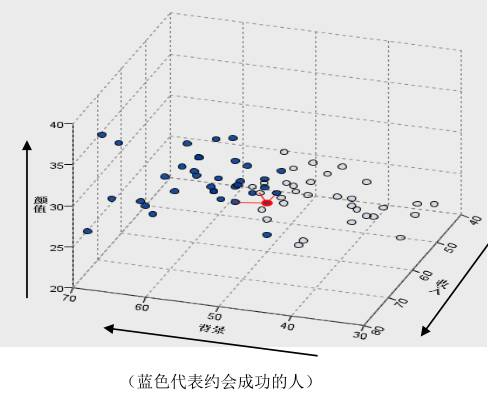KNN算法简介

1.     数据的前处理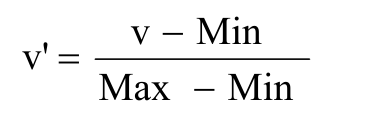2.     距离的计算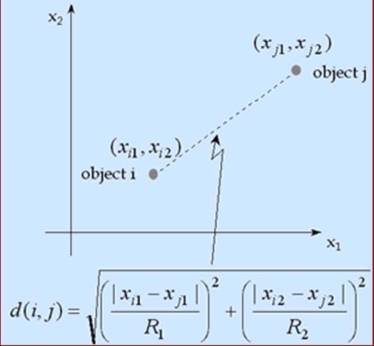3.     预测结果的推估

F指标（f1-score）：F指标 同时考虑Precision& Recall

1. 1.    读入数据集

2. 2.    描述性分析与探索性分析

3. 3.    KNN模型建立

4. 4.    模型的效果评估

%matplotlib inline

import os

import numpy as np

from scipy importstats

import pandas aspd

importsklearn.model_selection as cross_validation

importmatplotlib.pyplot as plt

import seaborn assns

import math

from scipy importstats,integrate

importstatsmodels.api as sm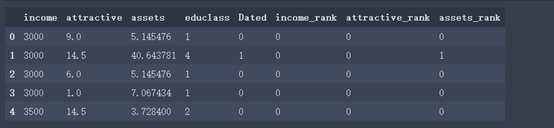orgData.info()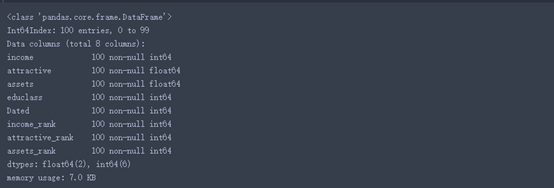orgData.describe()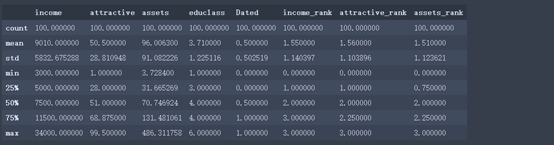Python的语法就是这么简洁到令人发指。从上述信息我们可以观察到各变量的计数、最大值、最小值、平均值等信息。以income为例，平均值为9010元，中位数为7500元。我们猜想是收入被平均了，如何更直观的看到呢？很简单，我们画个直方图。

sns.distplot(orgData['income'],fit=stats.norm);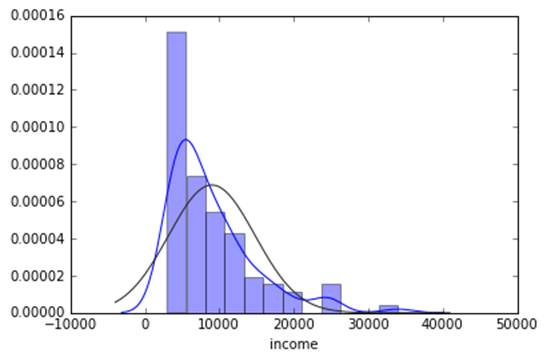sns.barplot(x='educlass',y='Dated',data=orgData);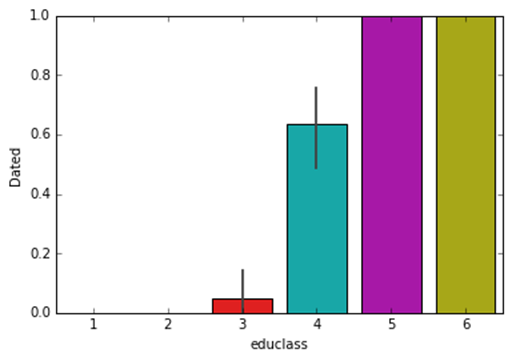fig,(axis1,axis2,axis3,axis4) = plt.subplots(1,4,figsize=(15,5))

sns.countplot(x='Dated',hue="educlass", data=orgData, order=[1,0], ax=axis1)

sns.countplot(x='Dated',hue="income_rank", data=orgData, order=[1,0], ax=axis2)

sns.countplot(x='Dated',hue="attractive_rank", data=orgData, order=[1,0], ax=axis3)

sns.countplot(x='Dated',hue="assets_rank", data=orgData, order=[1,0], ax=axis4)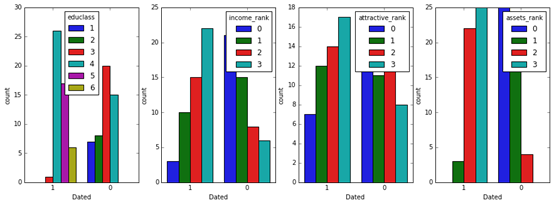X = orgData.ix[:,:4]

Y =orgData[['Dated']]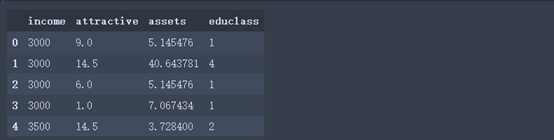from sklearnimport preprocessing

min_max_scaler = preprocessing.MinMaxScaler()

X_scaled =min_max_scaler.fit_transform(X)

X_scaled[1:5]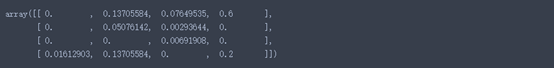#划分训练集和测试集

train_data,test_data, train_target, test_target = cross_validation.train_test_split(

X_scaled, Y, test_size=0.2, train_size=0.8,random_state=123)

fromsklearn.neighbors import KNeighborsClassifier

model =KNeighborsClassifier(n_neighbors=3)  # 默认欧氏距离

model.fit(train_data,train_target.values.flatten())

test_est =model.predict(test_data)

import sklearn.metricsas metrics

print(metrics.confusion_matrix(test_target,test_est, labels=[0, 1]))  # 混淆矩阵

print(metrics.classification_report(test_target,test_est))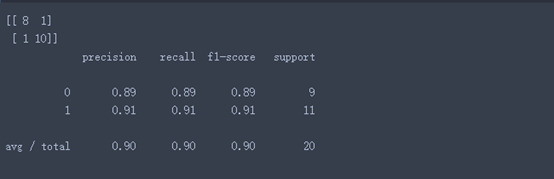【后话】当然，这里面只是用了一个简单的数据集去实操了一下KNN的做法，操作和语法都比较简单易用理解，同时遍历了一下我们数据挖掘的流程，相应的知识及后续的知识没有做过多的展开，比如前端的数据如何清洗，KNN中K值如何设定和交叉验证，使用朴素贝叶斯预测模型的准确率，特征选择，模型融合等。希望大家能有所收获。

~ From CDA学员

+ 关注

corcosa 13689人浏览